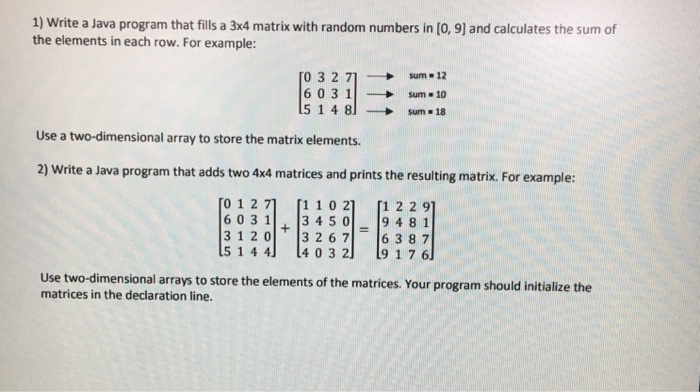Write a Java program that fills a 3×4 matrix with random numbers in [0, 9] and calculates the sum of the elements in each row.
For example: 0 3 2 7 1 →sum =12
6 0 3 1    →sum =12
5 1 4 8] → sum =18
Use a two-dimensional array to store the matrix elements.
2) Write a Java program that adds two 4×4 matrices and prints the resulting matrix.
For example: [0 1 27 [1 10 2 [1 2 2 9 3120 3 267 6 3 8 7 5 1 44 4 03 2 9 1 7 6 Use two-dimensional arrays to store the elements of the matrices.
Your program should initialize the matrices in the declaration line.